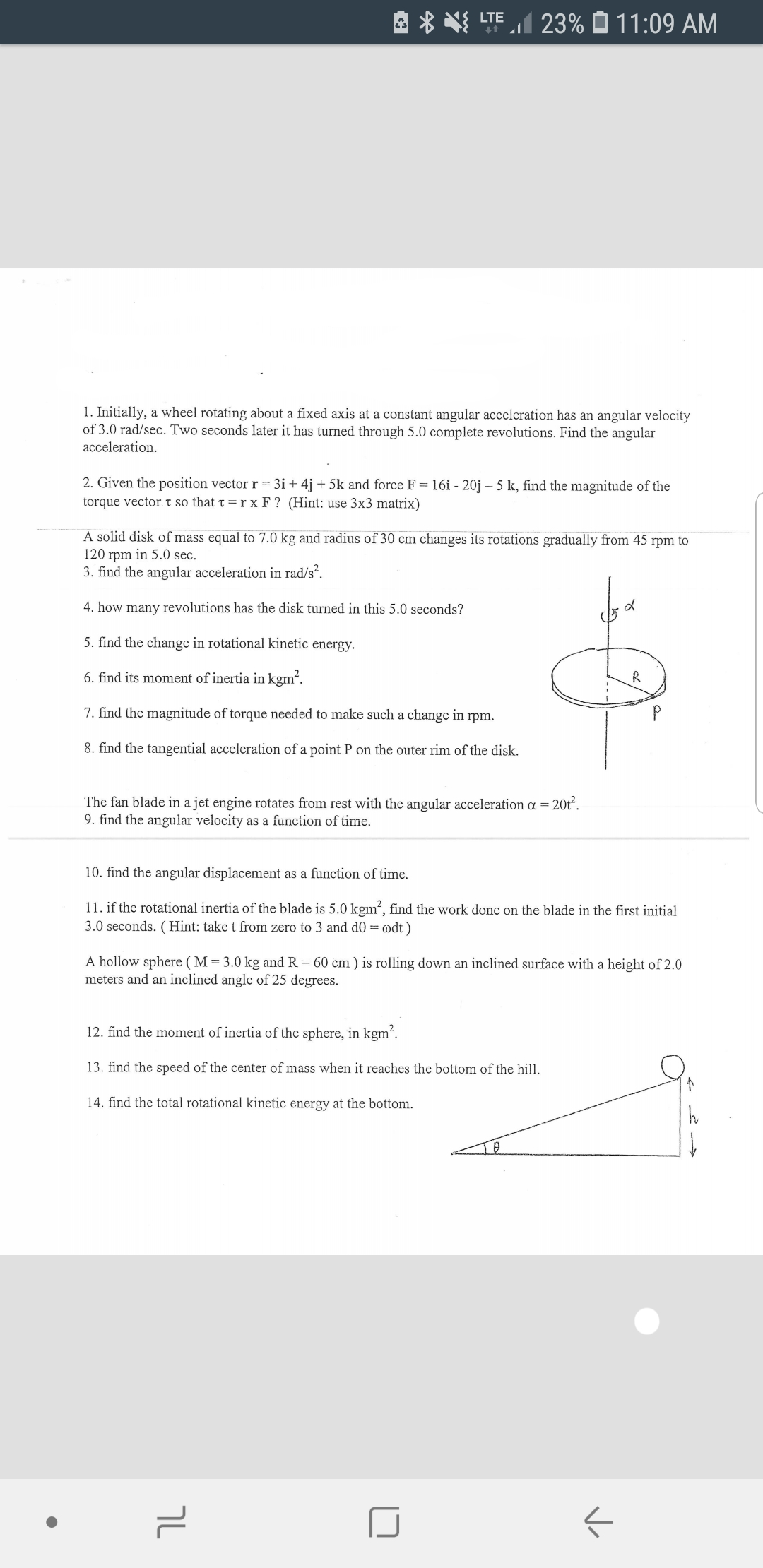# 23% 11: 09 AMLTE1. Initially, a wheel rotating about a fixed axis at a constant angular acceleration has an angular velocityof 3.0 rad/sec. Two seconds later it has turned through 5.0 complete revolutions. Find the angularacceleration2. Given the position vector r= 3i + 4j +5k and force F = 16i - 20j - 5 k, find the magnitude of thetorque vectort so that t = r x F ? (Hint: use 3x3 matrix)A solid disk of mass equal to 7.0 kg and radius of 30 cm changes its rotations gradually from 45 rpm to120 rpm in 5.0 sec3. find the angular acceleration in rad/s.4. how many revolutions has the disk turned in this 5.0 seconds?5. find the change in rotational kinetic energy6. find its moment of inertia in kgm27. find the magnitude of torque needed to make such a change in rpm.8. find the tangential acceleration of a point P on the outer rim of the disk.20t2The fan blade in a jet engine rotates from rest with the angular acceleration a =9. find the angular velocity as a function of time.10. find the angular displacement as a function of time.11. if the rotational inertia of the blade is 5.0 kgm2, find the work done on the blade in the first initial3.0 seconds. (Hint: take t from zero to 3 and d0 = odt)A hollow sphere ( M = 3.0 kg and R = 60 cm ) is rolling down an inclined surface with a height of 2.0meters and an inclined angle of 25 degrees.12. find the moment of inertia of the sphere, in kgm213. find the speed of the center of mass when it reaches the bottom of the hill14. find the total rotational kinetic energy at the bottomhך

Question
6 views

Part 12-14help_outlineImage Transcriptionclose23% 11: 09 AM LTE 1. Initially, a wheel rotating about a fixed axis at a constant angular acceleration has an angular velocity of 3.0 rad/sec. Two seconds later it has turned through 5.0 complete revolutions. Find the angular acceleration 2. Given the position vector r= 3i + 4j +5k and force F = 16i - 20j - 5 k, find the magnitude of the torque vectort so that t = r x F ? (Hint: use 3x3 matrix) A solid disk of mass equal to 7.0 kg and radius of 30 cm changes its rotations gradually from 45 rpm to 120 rpm in 5.0 sec 3. find the angular acceleration in rad/s. 4. how many revolutions has the disk turned in this 5.0 seconds? 5. find the change in rotational kinetic energy 6. find its moment of inertia in kgm2 7. find the magnitude of torque needed to make such a change in rpm. 8. find the tangential acceleration of a point P on the outer rim of the disk. 20t2 The fan blade in a jet engine rotates from rest with the angular acceleration a = 9. find the angular velocity as a function of time. 10. find the angular displacement as a function of time. 11. if the rotational inertia of the blade is 5.0 kgm2, find the work done on the blade in the first initial 3.0 seconds. (Hint: take t from zero to 3 and d0 = odt) A hollow sphere ( M = 3.0 kg and R = 60 cm ) is rolling down an inclined surface with a height of 2.0 meters and an inclined angle of 25 degrees. 12. find the moment of inertia of the sphere, in kgm2 13. find the speed of the center of mass when it reaches the bottom of the hill 14. find the total rotational kinetic energy at the bottom h ך fullscreen
check_circle

Step 1
1. Write the expression for moment of inertia of the sphere

Step 2

Substitute the values

Step 3
1. Write the expression for conservation...

### Want to see the full answer?

See Solution

#### Want to see this answer and more?

Solutions are written by subject experts who are available 24/7. Questions are typically answered within 1 hour.*

See Solution
*Response times may vary by subject and question.
Tagged in

### Rotational Mechanics